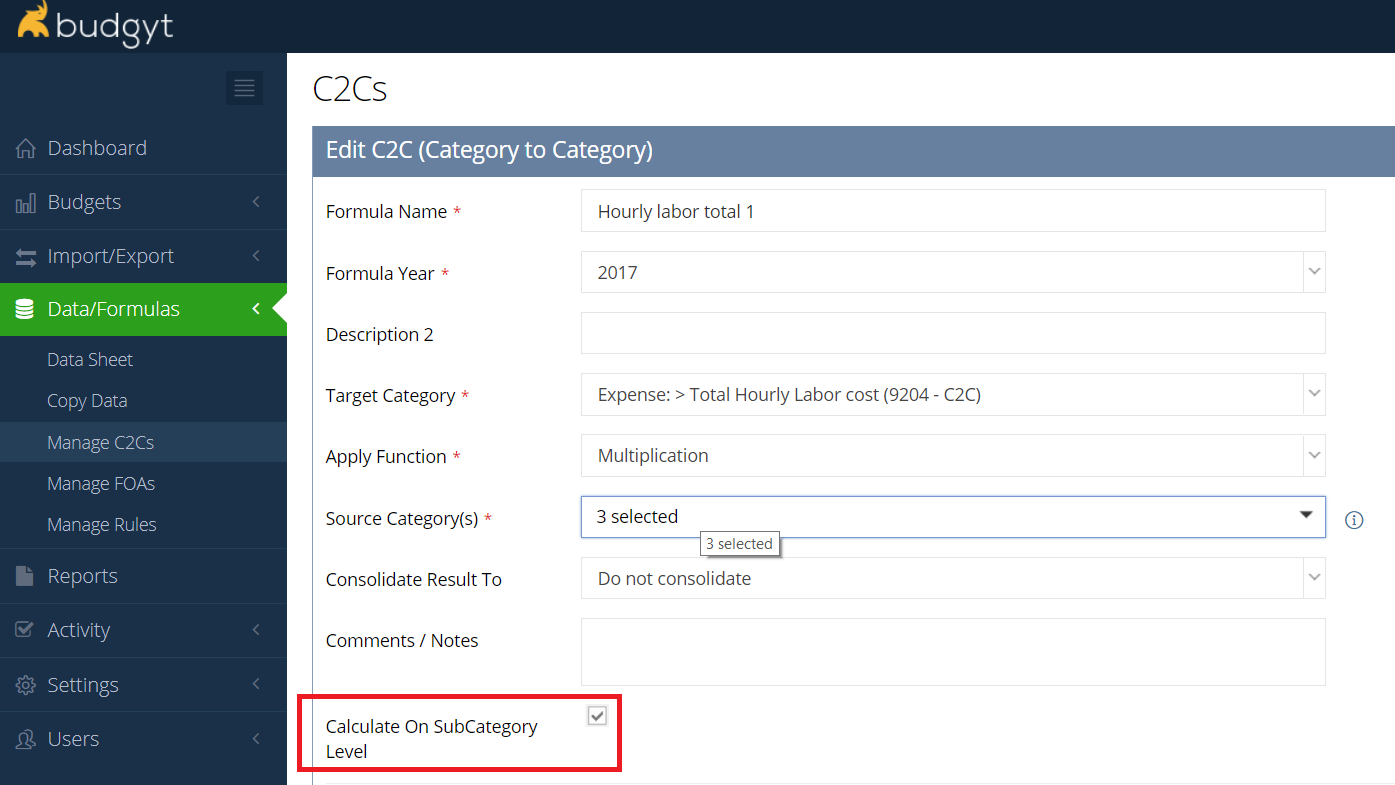Community Knowledge Base

# On the Add New C2C page, there is a check box for calculating at Sub Category level. What does this do?

Within Budgyt, we can create numerous sub categories in any of our categories. Sometimes it is useful to be able to calculate formulas based on those sub category entries rather than on the category itself.

For instance, you might have a category for Employees' Hours, where each employee is a sub category and the data represents hours worked by each of them. And you might also have a category for Hourly Rate by Employee, where each employee is again a sub category, and the data here represents the different hourly rates.

Creating a C2C where you multiply the 2 categories at the Sub Category level will multiply the first sub category line in the Hours category by the first in the Rates category, the 2nd by the 2nd etc etc, and the target category will show a total of all the individual multiplication calculations (the individual results will not be shown, only their sum).